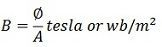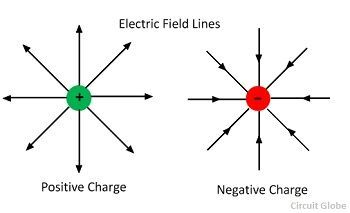# Difference between Electric & Magnetic Field

The one of the major difference between the magnetic and electric field is that the electric field induces around the static charge particle which is either negative or positive, whereas the magnetic field produces around the poles (i.e., the north and south pole) of the magnet. Some other differences between them are explained below in the form of comparison chart

## Comparison Chart

Basis For ComparisonElectric FieldMagnetic Field
DefinitionIt is the force around the electrical charge particle. The region around the magnetic where poles exhibits a force of attraction or repulsion.
UnitVolt/meter or Newton/coulomb Tesla, (Newton × Second) /(Coulomb × Meter)
SymbolEB
FormulaMeasuring InstrumentMagnetometer Electrometer
PoleMono pole Dipole
Electromagnetic FieldIt is perpendicular to the magnetic field. It is perpendicular to the electric field.
FieldVectorVector
Field LineInduces on a positive charge and terminate on a negative charge Generate at north pole and terminate at the south pole.
LoopElectric field lines do not form a closed loop.Magnetic line forms a closed loop.
Type of chargeNegative or positive charge. North or south pole.
ForceRepulsion force on like charges and attraction force on unlike charges.Repulsion force on like poles and attraction force on unlike poles.
DimensionExist in two dimensions Remain in three dimensions
WorkField can do work (the speed and direction of particles changes)Magnetic field cannot do work (speed of particles remain constant)

## Definition of Magnetic Field

The region around the magnetic where its poles exhibit a force of attraction or repulsion is called a magnetic field. The magnetic field also induces when the electric charges are moving in space or an electrical conductor.The moving charge carrier and magnet produce magnetic flux lines, and these lines are called magnetic field lines. It is a vector quantity because it has both magnitude and direction. The symbol B expresses the magnetic field, and it is measured in Tesla or Newton per meter.

## Definition of Electric Field

The force around the electrical charge particle is called an electrical field or electric field intensity. In other words, it is an area around the electric field where the force line exists. It has magnitude and direction. Hence it is a vector quantity.The symbol E expresses the electric field, and it is measured in newton/coulomb.

###Key Differences Between Electric Field and Magnetic Field

1. The region around the electric charge where the electric force exists is called an electric field. The area around the magnet where the pole of the magnet exhibits a force of attraction or repulsion is called a magnetic field.
2. The SI unit of an electric field is the Newton/coulomb, whereas the SI unit of magnetic field is Tesla.
3. Direction and magnitude specify the magnetic field. Thus it is a vector quantity. The electric field is also called a vector field.
4. The electric field intensity is measured by an electrometer whereas the magnetometer measures the magnetic field intensity.
5. In an electromagnetic field, the electric field is perpendicular to the magnetic field, whereas the magnetic field is perpendicular to an electric field.
6. The electric field produces by a unit pole charge, i.e., either by a positive or through a negative charge, whereas the magnetic field caused by a dipole of the magnet (i.e., the north and south pole).
7. The electric field line induces on a positive charge and extinguishes on a negative charge, whereas the magnetic field line generates from a north pole and terminate to the south pole of the magnet.
8. The electric field lines do not form a loop whereas the magnetic field lines form a closed loop.
9. The electric field is directly proportional to the flux whereas the intensity of the magnetic field depends on the number of field lines produces by the magnet.
10. In the electric field, the like charges repel each other, and the unlike charges attract each other, whereas in a  magnetic field the like poles repel each other and the unlike poles attract each other.
11. The electric fields induced by a single charge (positive or negative charge) whereas the magnetic fields induced by a north and south pole of the magnet.
12. The electric field intensity is expressed by the symbol E whereas the magnetic field intensity is expressed by B.
13. The electric field lines are measured in two dimensions, whereas the magnetic field lines are measured in three dimensions.
14. The electric field line can do work, i.e., the speed and the direction of the charge changes, whereas the magnetic field cannot do work, i.e., the direction of charge change but the speed of the particles remain constant.

### Conclusion:

The charge induces the electric field, and the magnetic field induces because of the north and south pole of the magnet.